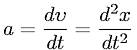Equations > Physics > Mechanics > Instantaneous Acceleration

### Instantaneous AccelerationLatex Code:

MathML Code:

 $a=\fracd\upsilon \mathrm{dt}=\frac{d}^{2}x{\mathrm{dt}}^{2}$

MathType 5.0: